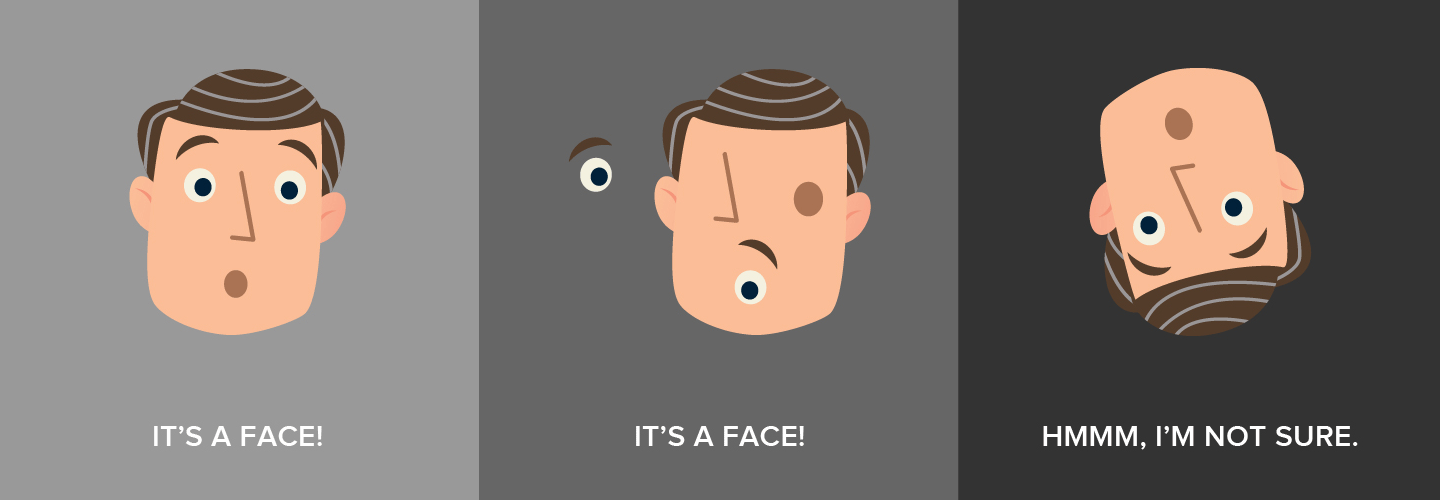/  Deep Learning Interview questions and answers   /  What is the difference between Convolutional Neural Network and Capsule Neural Network?## What is the difference between Convolutional Neural Network and Capsule Neural Network?

Ans: Convolutional Neural Network is used for the Image recognition by using different layers. Convolutional layer comprises set of independent filters where each filter is convolved with image. Pooling layer is used to reduce the spatial size of representation to reduce the number of parameters and computation in Neural Network. RELU is just a non-linearity which is applied similar to neural networks.

Drawback of convolutional neural network is explained in below figure

The Fully Connected layer is the layer of neurons which is at the end of CNN.

The main drawback of Convolutional Neural Network is it cannot identify the correct pattern of features in an object. To overcome this limitation of Convolutional Neural Network, Capsule Neural Network are introduced. These capsules correctly identify the image even when the image is rotated. All capsules in the first layer predict the output of capsules in next layer. Once the capsules in main layer figure out the capsules in the second layer to which it belongs, then those capsules in the main layer should be routed only to the corresponding capsule in the second layer.